﻿ Cyclic Voltammetry - A Versatile Electrochemical Method Investigating Electron Transfer Processes

### Cyclic Voltammetry - A Versatile Electrochemical Method Investigating Electron Transfer Processes

N. Aristov, A. Habekost

World Journal of Chemical Education

## Cyclic Voltammetry - A Versatile Electrochemical Method Investigating Electron Transfer Processes

N. Aristov1, A. Habekost1,1Department of Chemistry, University of Education Ludwigsburg, Reuteallee, Ludwigsburg, Germany

### Abstract

Three experiments are presented to introduce students to the capabilities of cyclic voltammetry (CV) for finding redox couples suitable for, e.g., battery development. The systems chosen involve only one-electron transfer, but already display complex behaviours that can be delineated with CV as being reversible, quasi-reversible, or irreversible, and the rate constants for the electron transfer can be estimated by the theory of Nicholson and Shain.

• N. Aristov, A. Habekost. Cyclic Voltammetry - A Versatile Electrochemical Method Investigating Electron Transfer Processes. World Journal of Chemical Education. Vol. 3, No. 5, 2015, pp 115-119. http://pubs.sciepub.com/wjce/3/5/2
• Aristov, N., and A. Habekost. "Cyclic Voltammetry - A Versatile Electrochemical Method Investigating Electron Transfer Processes." World Journal of Chemical Education 3.5 (2015): 115-119.
• Aristov, N. , & Habekost, A. (2015). Cyclic Voltammetry - A Versatile Electrochemical Method Investigating Electron Transfer Processes. World Journal of Chemical Education, 3(5), 115-119.
• Aristov, N., and A. Habekost. "Cyclic Voltammetry - A Versatile Electrochemical Method Investigating Electron Transfer Processes." World Journal of Chemical Education 3, no. 5 (2015): 115-119.

 Import into BibTeX Import into EndNote Import into RefMan Import into RefWorks

12
Prev Next

### 1. Introduction

It is no secret that the need to switch from petroleum-based, CO2-producing energy sources to alternatives, in particular those based on electrochemical principles, is ever more pressing. For that reason alone, this topic is an important goal of general undergraduate instruction in chemistry. One of the most important experimental methods to investigate redoxsystems for using as rechargeables is cyclic voltammetry (CV).

The experimental CV results are sometimes difficult to interpret, given the plethora of possible processes (Table 1), and also the need to consider the diffusion of the reacting species to and from the electrode surface on which the reaction takes place.

Nevertheless, there are systems that lend themselves as subjects for an introduction to the use of CV in understanding electrochemical processes.

Therefore, in this paper we present and interpret three relative simple but instructive systems to understand the principles of CV, a method in electrochemistry for determining electrode reaction mechanisms, standard electron transfer rate constants, and also diffusion coefficients. We propose and describe procedures that show the behavior of reversible, quasi-reversible and irreversible systems (the first two are necessary condition for rechargeables), restricting the discussion to only the first case in Table 1, that of simple electron transfer. On base of the theory of Matsuda and Ayabe  and Nicholson and Shain  the experimenter can calculate the electron transfer rate constant, the decisive factor describing the electrode process, from the CV in a very simple way.

### 2. Some Features of Cyclic Voltammetry

Many texts on CV are available [see, e.g., 3-10]. Here we will only present a basic outline that is necessary to understand the presented experiments.

A CV is obtained by measuring the current between the working and the counter electrode as a function of the potential (normalized to the potential of the reference electrode). To do this, the experimenter uses a three-electron setup and varies the potential of an electrode (the “working” electrode), which is immersed in an unstirred solution, and measures the resulting current.

A triangular potential sweeps the potential of the working electrode between the starting potential to the switching potential and back again. The scan rate v (in mV/s) is an important parameter, as will be shown below.

The current flows in or out of the working electrode to or from a counter electrode. The potential of the working electrode is controlled versus a reference electrode, e.g., a saturated calomel or a silver/silver chloride electrode. The reference electrode passes no current.

All these requirements can be fulfilled by a potentiostat.

The rate of an electron transferred between the electrode and the solution depends on the potential.

Here are the main points to consider when starting out with CV:

•  According to the IUPAC recommendation anodic peaks point upward, cathodic downward.

•  The standard redox potential E0 can be calculated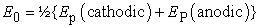(1)

where EP are the peak potentials.

•  If the experimenter wants to determine the standard potential E0 the scan rate should be slowed down to minimize ΔEp. However, there is a lower limit to the scan rate: This is set by the ability to maintain convection-free conditions. This means that scan rates lower than 1 mV/s are often not useful. (Calculation of E0 requires the potential of the reference electrode to compare E0 with tabulated values which are relative to the normal hydrogen electrode NHE).

•  If the current peaks appear to be sliding apart as a function of scan rate, the process is quasi-reversible (or, in an extreme case, irreversible).

•  If an organic, high resistance electrolyte is used, the applied potential is not identical with the theoretical potential between the working and reference electrodes. The resistance of the solution causes a so-called IR-drop. This means, that the potential of the working electrode drifts away with I∙R and is unreliable. For this reason a highly conducting supporting electrolyte, which is not electroactive in the potential range being studied, is added to the solution. In the experiments described here this is not necessary because the electrolyte itself is highly conductive.

Two qualities, parameterized by the standard electron rate constant k0 and the mass transport to or from the working electrode mtransport, determine the CV. For a derivation of these parameters we refer readers to, e.g., Ref . We limit ourselves here to a qualitative description that will allow an initial interpretation of the results.

The electron transfer rate constant for a reduction and oxidation process is a function of the applied potential and can be described as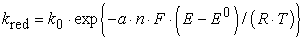(2)

and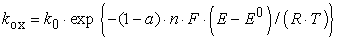(3)

where k0 is the standard electron-transfer rate constant in cm/s at the standard potential E0, a is the so-called transfer coefficient (a measure of the symmetry of the activation energy barrier for the oxidation and reduction processes), n is the number of the transferred electrons, F is the Faraday constant, E the applied potential, R the ideal gas constant, and T the absolute temperature.

The exponential dependence of k on the potential E results in a steep rise in the current. This leads to a depletion of the concentration of the corresponding species at the electrode. Now, diffusion is the only process in an unstirred solution by which the reactant can move to the electrode surface. (Migration is mainly controlled by the electrolyte and not by the electroactive substances). As diffusion is slow (diffusion coefficients are in the range of 10-5 cm2/s) the current does not increase exponentially with E as (2) and (3) indicate but decreases after the species reacted on the electrode surface and so a depletion layer results.

The mass transport is given by eq. (5):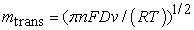(4)

In each case the slowest process determines the electrochemical behavior:

•  If k0 >> mtrans then the electrode process is reversible and diffusion controlled.

•  In the intermediate, the so-called quasi-reversible case, diffusion and electron transfer are in the same order (k0 ≈ mtrans).

•  The process will be rate-determined if the mass transport is faster than the electron transfer (k0<<mtrans) and the electron transfer is irreversible.

One should note here that electrochemical and chemical reversibility are different things: chemical irreversibility means that one of the redox partners is removed from the electrode by chemical reaction, electrochemical irreversibility means that the electron transfer is hindered.

With the help of the theory of Matsuda and Ayabe and Nicholson and Shain, who introduced the dimensionless parameter Λ the relation between the standard rate constant k0 and mass transport mtrans, one can estimate the transition between reversible and irreversible electrochemical reactions: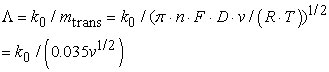(5)

for D = 10-5 cm2/s, n= 1, T = 293 K and v in V/s.

In the irreversible and quasi-reversible cases the theoretical modeling of systems is quite complex. Matsuda and Ayabe followed by Nicholson and Shain have devised a way of gleaning estimates of the k0 rate constant from the difference between the peak potentials ΔEp via Λ.

Discussing the derivation of the correlation between ΔEp and Λis beyond the scope of this paper.

#### Table 2. Correlation between the Nicholson parameter ΔEp and Λ

In Table 2 (from Ref. ) some values are provided so that readers may be able to interpret their own data.

The experimenter can thus distinguish between reversible, quasi-reversible and irreversible electron transfer by inspecting ΔEP: First, one has to measure ΔEP in the CV, then calculate Λ from Table 2 and compare with the data of Table 3.

#### Table 3. Correlation between Nicholson parameter and k0 to determine the electron transfer mechanism 

There are three other clues that a cyclovoltammogram provides that indicate that the observed process is reversible.

The first is that the peak currents Ip for the forward and reverse reactions are the same,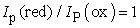(6)

The second is that the peak current is proportional to the square root of the scan rate: Reversible processes show a v1/2-dependence of their current peaks according to the Randles-Sevcik equation: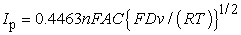(7)

Ip = current maximum in amps, n = number of electrons transferred in the redox event, A = electrode area in cm2, F = Faraday constant in C mol−1, D = diffusion coefficient in cm2/s , C = concentration in mol/cm3, ν = scan rate in V/s, R = g in VC K−1 mol−1, T= temperature in K.

Third, the difference between the electrode potentials at which reduction and oxidation occur is constant at all scan rates: If the positions of the maximum current peaks with regard to their potential do not change as a function of the scan rate, and the heights of the anodic and cathodic peaks appear to be equal, then the process occurring is reversible. If the peaks are about 59 mV apart then the process is a reversible one-electron transfer (n = 1):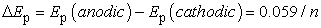(8)

With eq. (8) the experimenter can calculate the number of transferred electrons n.

These relationships can be derived theoretically [1, 2, 3].

In the quasi-reversible and the (electrochemical) irreversible case one can see that the current peaks are drifting apart with increasing scan rate up to hundreds of millivolts. Now the kinetic process, i.e. the electron transfer, is determining the electrochemical behavior.

In cyclic voltammetry the scan rate is a means that provides the experimenter with a tool to control the electrochemical process either by electron transfer process or by mass transport: By varying the scan rate we vary the diffusion layer thickness: at slow scan rates, the diffusion layer is thick, while at faster scan rates the diffusion layer is thinner. Since the electrochemical process reflects the competition between the electrode reaction and the diffusion, faster scan rates will favor electrochemical irreversibility (controlled by the electron transfer rate) and the peak potentials will drift apart.

The objectives of the following CV experiments are to examine the CV as a function of the scan rate to determine whether the electron transfer is reversible, quasi-reversible, or irreversible.

### 3. Experiments

3.1. Experimental procedures

The experiments can be completed by a pair of students within 8 hours of laboratory work.

The CVs shown here were recorded with a μStat 400 potentiostat from DropSens, distributed by Metrohm. Drops of the appropriate solutions were placed on the electrodes with pipettes; the substances examined as well as the corresponding concentrations are given for the various experiments.

The experiments were performed with two different screen printed electrodes: DS 550 (working and counter electrode, Pt, reference electrode, Ag) and DS 110 (working and counter electrode, graphite, reference electrode, Ag). The reference potential of the silver electrode is a pseudo-potential. That means that the silver reacts not much with an aqueous solution but enough to form a low quantity of silver ions. These ions will react with the supporting electrolytes which, in our experiments, contain sulfate to form insoluble silver sulfate. Therefore, the reference potential is approximately defined.

Figure 1, Figure 3 and Figure 4 show CVs of reversible, quasi-reversible, and irreversible processes. We’d like to point out an additional item in Figure 4 that shows two different CVs, one quasi-reversible, the other irreversible. The difference between these two systems was not the solution, but the electrode – the redox is quasi-reversible on platinum and irreversible on graphite.Download asVeiw figureFigures index
Figure 1. CV of the reversible redox of Ru (III) – Ru (II) on a platinum working electrode (DS 550). Scan rates: 10 – 60 mV/s. The slower scan rates yield lower currents. The arrow indicates increasing scan rate, the bars indicate the calculation of Ip(anodic) and Ip(cathodic)
3.2. Reversible Reaction 

Chemicals and procedure

3 mg hexammineruthenium chloride (Sigma Aldrich 262005) in 10 mL 0.1 M aqueous Na2SO4 (corresponds to 1/1000 mol hexammineruthenium chloride).

Here we describe the measurement procedure with the DropSens-potentiostat. We think that the procedure is similar to other potentiostats.

First, plug in the screen printed electrodes into the potentiostat with the appropriate cable.

Drop the solution onto the screen printed electrodes so that all three electrodes are coated.

Start the CV program and define the scan rate, the increment of the scan, the number of the scans, and the starting, the switching and the final potential.

Here we take five different scan rates (10 – 60 mV/s) and the scan range: -0.3 V à 0.5 V à -0.3 V

Hazard: No hazardous chemicals.

The peak potentials do not drift apart as a function of the scan rate and Ip (cathodic) ≈ Ip (anodic).

The difference between the peak potential is about 60 mV, indicating a one-electron-process. According to Table 3 the Nicolson parameter Λ =19 and therefore k0 > 19·0.035·v1/2 > 0.67·v1/2 and with v = 0.06 V/s k0 > 0.16 cm/s.

The data in Figure 2 are plotted according to the Randles-Sevcik (eq. 8). The graph yields the linear relationship indicative of Ip ˜ v1/2.Download asVeiw figureFigures index
Figure 2. Randles-Sevcik plot Ip(anodic) vs. v1/2

The Randles-Sevcik plot verifies the linearity between the peak current and the square root of the scan rate indicating reversible electron transfer (the analogous behavior of the cathodic peak is not shown here).

3.3. Quasi-reversible reaction 

Chemicals and procedure

4 mg potassium hexacyanoferrate (III) (SigmaAldrich, 31254) in 10 mL 0.1 M aqueous Na2SO4 (corresponds to 1/1000 mol potassium hexacyanoferrate (III)).

Five different scan rates (2, 5, 10, 20, 50 mV/s), scan range: 0.5 V à -0.3 V à 0.5 V

Hazard: No hazardous chemicals.

The currents peaks for the oxidation and reduction slide apart with increasing scan rate from 75 mV at 2 mV/s to 160 mV at 50 mV/s.

Using the theory of Nicholson and Shain we find that the values of Λ change from 1.51 to 0.185 with increasing scan rate.

In Table 4 we estimate k0 using the Nicholson and Shain method. The average value is 2.2∙10-3 cm/s (for D ≈ 10-5 cm2/s).

#### Table 4. Derivation of k0 for Fe(II) / Fe(III) from ΔEpDownload asVeiw figureFigures index
Figure 3. Quasi-reversible redox of Fe (II) / Fe (III) on a platinum working electrode (DS 550). Scan rates: 2, 5, 10, 20, 50 mV/s, lower scan rate yields lower current
3.4. Quasi-reversible and Irreversible Reaction – Different Electrodes [14,15]

Chemicals and procedure

0.32 mg N,N,N’,N’-tetramethyl-p-phenylenediamine (TMPD, Wurster’s Blue, SigmaAldrich, T 7394) in 10 mL 2M H2SO4 (corresponds to 1/500 mol TMPD). Scan rate 20 – 50 mV/s.

Hazard: No hazardous chemicals.Download asVeiw figureFigures index
Figure 4. CV of tetramethyl-p-phenylenediamine. Black curve: DS 550 Pt electrode. Scan rates: 20, 30, 40, 50 mV/s yielding ΔEp 110 mV – 160 mV: quasi-reversible. Blue curve: DS 110 graphite electrode. Scan rates: 20, 30, 40, 50 mV/s yielding ΔEp 250 mV – 350 mV

Electron transfer in TMPD can be quasi-reversible or irreversible depending on the electrode used (Figure 4). Here we measured a peak current drift for Pt of 110 mV to 160 mV (scan rate 20 - 50 mV/s) yielding k0 ≈ 10-3cm/s, whereas for graphite it is between 250 mV and 350 mV (scan rate 20 - 50 mV/s) yielding ko << 10-4cm/s. DTMPD ≈ 6∙10-6cm2/s in n-butanol  and is thus within in the range of the assumed value in Table 3.

### References

  Matsuda, H., Ayabe, Y., The theory of the cathode-ray polarography of Randles-Sevcik, Zeitschrift fuer Elektrochmie and Angewandte Physikalische Chemie. 1955, 59, 494-503.In article  Nicholson, R. S., Shain, I., Theory of Stationary Electrode Polarography Single Scan and Cyclic Methods Applied to Reversible, Irreversible, and Kinetic Systems, Adv. Anal. Chem. 1964, 36(4), 706-723.In article View Article  Bard, A. J., Faulkner, L. R., Electrochemical Methods: Fundamentals and Applications (Chemistry), Wiley and Sons, 2001.In article  Gosser, Jr, D. K., Cyclic Voltammetry. Simulation and Analysis of Reaction Mechanism, VCH, Weinheim, Germany, 1993.In article  Fisher, A. C., Electrode Dymanics, Oxford Science Publication, New York, 2009.In article  Brett, C. M. A., Oliveira Brett, A. M., Electroanalysis, Oxford Science Publication, New York, 2005.In article  Compton, R. G., Banks, C. E., Understanding Voltammetry, 2nd Edition, Imperial College Press, 2011.In article View Article  Mabbott, G. A., An Introduction to Cyclic Voltammetry, J. Chem. Educ. 1983, 60, 607-702.In article View Article  Kissinger, P. T., Heineman, W. R., Cyclic Voltammetry, J. Chem. Educ. 1983, 60, 702-706.In article View Article  van Benschoten, J. J., Lewis, Y. T., Heineman, W. R., Roston, D. A., Kissinger, P. T., Cyclic Voltammetry Experiments, J. Chem. Educ. 1983, 60, 772-776.In article View Article  Heinze, J., Cyclovoltammetrie – Die “Spektroskopie” des Elektrochemikers, Angewandte Chemie. 1984, 96, 823-840.In article View Article  Lim, H.S, Barclay, D.J., Anson, F.C., Formal potentials and cyclic voltammetry of some ruthenium-ammine complexes, Inorg. Chem., 1972, 11, 1460-1466.In article View Article  Rock, P.A., The Standard Oxidation Potential of the Ferrocyanide-Ferricyanide Electrode at 25°C and the Entropy of Ferrocyanide Ion, J. Phys. Chem. 1966, 70, 576-580.In article View Article  Beiginejad, H., Nematollahi, D., Farmaghani, F., Electrochemical oxidation of some aminophenols in various pHs, J. Electrochem. Soc. 2013, 160, 41-46.In article View Article  Long, S., Silvester, D. S., Barnes, A. S., Rees, N. V., Aldous, L., Hardacre, C., Compton, R. G., Oxidation of several p-phenylenediamines in room temperature ionic liquids: estimation of transport and electrode kinetic parameters, J. Phys. Chem. C. 2008, 112, 6993-7000.In article View Article  Moressi, M. B., Zon, M. A., Fernandez, H., The determination of thermodynamic and heterogeneous kinetic parameters of the TMPD/TMPD•+ redox couple in tetrahydrofuran and n-butanol using ultramicroelectrodes. Quasi steady-state voltammetry, J. Braz. Chem. Soc. 1994, 5, 167-72.In article View Article